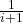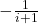# Tag Archives: MS1

## Betweenness

The last section is on “Miscellaneous” problems.  There are some weird ones in here, but we start with a pretty straightforward number problem.

The problem: Betweenness.  This is problem MS1 in the appendix.

The description: Given a set C of triples (a,b,c), where each element of each triple comes from a superset A, can we find an ordering of the elements of A such that according to that ordering, each triple is in either increasing or decreasing order?

Example: It’s actually hard to do a good example for this because most groups of symbols (digits, letters, subscripts, …) have an implicit ordering of elements, and to go against that would be confusing.  Having random symbols without connotations of meaning gets pretty confusing pretty quickly as well.

So let’s lean in and say A = {a,b,c,d,e,f} and our triples are:

(a,d,f)
(f,d,b)
(h,d,a)
(f,g,h)
(d,f,h)

We’re allowed any ordering of the elements of A, and the “normal” lexicographical one with ‘a’ first and ‘f’ last satisfies the betweenness rules.

But now suppose we add the new triple: (b,a,d).  That is an illegal triple in our normal order, but if we change our ordering so that ‘b’ is first and ‘a’ is second, we can still satisfy all of the triples.

Reduction: The paper by Opatrny uses Hypergraph 2-Colorability (which we called “Set Splitting”).  Recall that our reduction only made sets of size 3, so we can start with an instance where each set has at most 3 elements (or, each hyperedge connects at most 3 vertices).

So, suppose we have a set of N vertices s1..sn, a set of J 3-vertex hyperedges (ai, bi, ci), as well as M 2-vertex “regular” edges (di, ei).

Our first job is to build superset A.  It has:

• One symbol for each vertex,
• A new symbol X.
• A set of J new symbols Yk, one for each 3-vertex hyperedge.

Now we build C.

• Each hyperedge (ak, bk, ck) generates two triples: (ak, Yk, bk) and (Yk, X, ck)
• Each two-vertex edge (dk, ek) generates the triple (dk, X, ek)

Suppose the graph had a 2-coloring.  Recall that meant that each vertex had a color (the paper uses red and blue) so that each edge and hyperedge touches vertices of both colors.  We need to make an ordering of A that satisfies all triples.  The ordering will have positive,  negative, and rational ranks.

• X has rank of 0.
• If vertex si is red, its rank is i.  If it’s blue, its rank is -i.
• Each Yi was associated with a hyperedge (ai, bi, ci).
• If ai and bi have the same color (and so the same sign), Yi‘s rank is min(rank(ai), rank(bi)) +.
• If they have different colors, the rank isif ci is blue, andif ci is red

So, suppose every edge had 2 colors.  We know:

• If ai and bi are the same color, they are the same sign, and Yi is between them, satisfying the (ak, Yk, bk) triple.
• If ai and bi are the same color, ci must be the other color (and the other sign from Yi), satisfying the (Yk, X, ck) triple.
• If ai and bi are different colors, they are different signs.  Setting Yi to a value between 1 and -1 satisfies the (ak, Yk, bk) triple.
• If ai and bi are different colors, ci can be either color, so making Yi the opposite sign as ci satisfies the (Yk, X, ck) triple.
• An edge with just 2 vertices needs both vertices opposite colors, and thus opposite signs, so the (dk, X, ek) triple is satisfied.

So far, so good.  In the opposite direction, suppose we have a solution to the betweenness instance.  We’ll assign colors to vertices such that a vertex’s color is red if its rank is > X’s rank.  Otherwise, it will be assigned blue.  Our triples will now allow any set of ai, bi, and ci to all be greater than or less than X (or for both di and ei to be greater than or less than X), which means that every edge in the hypergraph has both a red and a blue vertex.

Difficulty: 7.  It’s relatively easy to see what you need to do.  It’s harder to make the numbers actually work.  Even this paper has to resort to using rational numbers for the ranks, which is a fixable problem, but certainly not obvious.